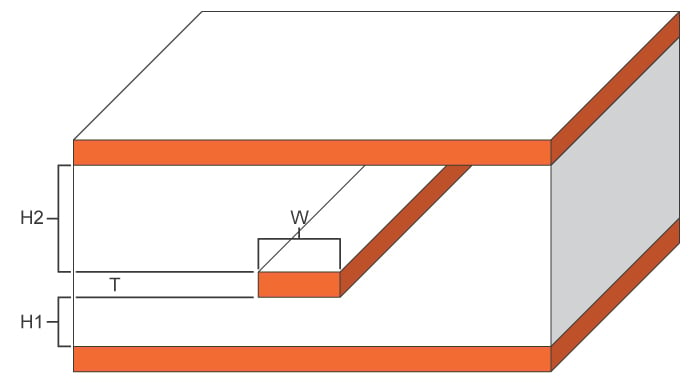# Asymmetric Stripline Impedance Calculator

## This calculator helps you compute the characteristic impedance of an asymmetric stripline

Ohms

### Overview

This calculator is designed to find the characteristic impedance of an asymmetric stripline - a flat conductor between two ground planes. Take note that this assumes that the distance between the conductor and the planes is not the same for both reference planes; hence the asymmetric term.

Just enter the given values for trace thickness, substrate heights, trace width and subtrate dielectric in the calculator above and press the "calculate" button. The default units for all given values, except the subtrate dielectric, is in mil, but it is possible to select other units.### Equations

$$Z_{0} = \frac{1}{\sqrt{\epsilon_{r}}}(Z_{0, ss} - \Delta Z_{0, air})$$

$$\Delta Z_{0,air} = 0.0325\pi (Z_{0,air})^{2}(0.5 - \frac{1}{2} \frac{2H_{1} + 1}{H_{1}+H_{2}+T})^{2.2} (\frac{T+W}{H_{1}+H_{2}+T})^{2.9}$$

$$Z_{0,air} = 2(\frac{Z_{0,ss1} Z_{0,ss2}}{Z_{0,ss1} + Z_{0,ss2}})$$

$$m = \frac{6 H_{eff}}{3 H_{eff} + T}$$

$$H_{eff} = \frac{H_{1}+H_{2}}{2}$$

Where:

$$Z_{0}$$ = characteristic impedance of the asymmetric stripline in ohms (Ω).

$$H_{1}$$ = subtrate height 1

$$H_{2}$$ = subtrate height 2

$$W$$ = trace width

$$T$$ = trace thickness

$$\epsilon_{r}$$ = substrate dielectric

$$Z_{0,air}$$ = characteristic impedance of air

$$Z_{0,ss1}$$ = characteristic impedance of symmetrical stripline with $$b=h_{1}$$ (see symmetric stripline tool)

$$Z_{0,ss2}$$ = characteristic impedance of symmetrical stripline with $$b=h_{2}$$ (see symmetric stripline tool)

### Applications

It is common to find asymmetric striplines in double-sided printed circuit boards where the distance from trace to plane above is not the same with the distance from trace to plane below. Many engineering designs involve such structure and so it is helpful to know how to model such impedance. If a model is determined, then designing a trace will not be such a hard task. The engineer can then design his own asymmetric stripline transmission line that meets his requirements.